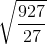# 1st PUC Chemistry Series-2

#### PUC Science 1st Year Chemistry Series-2 quiz

0
Created on Bywefru

1st PUC Chemistry Series 2

1 / 10

Q.11 A container with a pin-hole contains equal moles of H2(g) and O2(g). Find the fraction of oxygen gas escaped at the same time when one-fourth of hydrogen gas escapes

2 / 10

Q.12 What are the conditions for gas like Carbon monoxide to obey the ideal gas laws?

3 / 10

Q.13 If the temperature is doubled, the average velocity of a gaseous molecule increases by

4 / 10

Q.14 Find the molecular mass of a gas that takes three times more time to effuse as compared to He with the same volume

5 / 10

Q.15 At the same temperature, the average molar kinetic energy of N2 and CO is

6 / 10

Q.16 Find the temperature at which the rate of effusion of Nis 1.625 times to that of SO2 at 500℃

7 / 10

Q.17

Find the change in the root mean square speed of the gas on raising the temperature from 27℃ to 927℃8 / 10

Q.18  If 1.204 x 1021 molecules of H2SO4 are removed from 392 mg of H2SO4, find the moles of H2SO4 left.

9 / 10

Q.19 Find the fraction of the total pressure exerted by hydrogen if it is mixed with ethane in an empty container at 25℃

10 / 10

Q.20 Find the pH of a solution when 0.01 M HCl and 0.1 M NaOH are mixed in equal volumes